$\newcommand{\NN}{\mathbb{N}} \newcommand{\CC}{\mathbb{C}} \newcommand{\GG}{\mathbb{G}} \newcommand{\LL}{\mathbb{L}} \newcommand{\PP}{\mathbb{P}} \newcommand{\QQ}{\mathbb{Q}} \newcommand{\RR}{\mathbb{R}} \newcommand{\VV}{\mathbb{V}} \newcommand{\ZZ}{\mathbb{Z}} \newcommand{\FF}{\mathbb{F}} \newcommand{\KK}{\mathbb{K}} \newcommand{\UU}{\mathbb{U}} \newcommand{\EE}{\mathbb{E}} \newcommand{\Aa}{\mathcal{A}} \newcommand{\Bb}{\mathcal{B}} \newcommand{\Cc}{\mathcal{C}} \newcommand{\Dd}{\mathcal{D}} \newcommand{\Ee}{\mathcal{E}} \newcommand{\Ff}{\mathcal{F}} \newcommand{\Gg}{\mathcal{G}} \newcommand{\Hh}{\mathcal{H}} \newcommand{\Ii}{\mathcal{I}} \newcommand{\Jj}{\mathcal{J}} \newcommand{\Kk}{\mathcal{K}} \newcommand{\Ll}{\mathcal{L}} \newcommand{\Mm}{\mathcal{M}} \newcommand{\Nn}{\mathcal{N}} \newcommand{\Oo}{\mathcal{O}} \newcommand{\Pp}{\mathcal{P}} \newcommand{\Qq}{\mathcal{Q}} \newcommand{\Rr}{\mathcal{R}} \newcommand{\Ss}{\mathcal{S}} \newcommand{\Tt}{\mathcal{T}} \newcommand{\Uu}{\mathcal{U}} \newcommand{\Vv}{\mathcal{V}} \newcommand{\Ww}{\mathcal{W}} \newcommand{\Xx}{\mathcal{X}} \newcommand{\Yy}{\mathcal{Y}} \newcommand{\Zz}{\mathcal{Z}} \newcommand{\al}{\alpha} \newcommand{\la}{\lambda} \newcommand{\ga}{\gamma} \newcommand{\Ga}{\Gamma} \newcommand{\La}{\Lambda} \newcommand{\Si}{\Sigma} \newcommand{\si}{\sigma} \newcommand{\be}{\beta} \newcommand{\de}{\delta} \newcommand{\De}{\Delta} \renewcommand{\phi}{\varphi} \renewcommand{\th}{\theta} \newcommand{\om}{\omega} \newcommand{\Om}{\Omega} \renewcommand{\epsilon}{\varepsilon} \newcommand{\Calpha}{\mathrm{C}^\al} \newcommand{\Cbeta}{\mathrm{C}^\be} \newcommand{\Cal}{\text{C}^\al} \newcommand{\Cdeux}{\text{C}^{2}} \newcommand{\Cun}{\text{C}^{1}} \newcommand{\Calt}{\text{C}^{#1}} \newcommand{\lun}{\ell^1} \newcommand{\ldeux}{\ell^2} \newcommand{\linf}{\ell^\infty} \newcommand{\ldeuxj}{{\ldeux_j}} \newcommand{\Lun}{\text{\upshape L}^1} \newcommand{\Ldeux}{\text{\upshape L}^2} \newcommand{\Lp}{\text{\upshape L}^p} \newcommand{\Lq}{\text{\upshape L}^q} \newcommand{\Linf}{\text{\upshape L}^\infty} \newcommand{\lzero}{\ell^0} \newcommand{\lp}{\ell^p} \renewcommand{\d}{\ins{d}} \newcommand{\Grad}{\text{Grad}} \newcommand{\grad}{\text{grad}} \renewcommand{\div}{\text{div}} \newcommand{\diag}{\text{diag}} \newcommand{\pd}{ \frac{ \partial #1}{\partial #2} } \newcommand{\pdd}{ \frac{ \partial^2 #1}{\partial #2^2} } \newcommand{\dotp}{\langle #1,\,#2\rangle} \newcommand{\norm}{|\!| #1 |\!|} \newcommand{\normi}{\norm{#1}_{\infty}} \newcommand{\normu}{\norm{#1}_{1}} \newcommand{\normz}{\norm{#1}_{0}} \newcommand{\abs}{\vert #1 \vert} \newcommand{\argmin}{\text{argmin}} \newcommand{\argmax}{\text{argmax}} \newcommand{\uargmin}{\underset{#1}{\argmin}\;} \newcommand{\uargmax}{\underset{#1}{\argmax}\;} \newcommand{\umin}{\underset{#1}{\min}\;} \newcommand{\umax}{\underset{#1}{\max}\;} \newcommand{\pa}{\left( #1 \right)} \newcommand{\choice}{ \left\{ \begin{array}{l} #1 \end{array} \right. } \newcommand{\enscond}{ \left\{ #1 \;:\; #2 \right\} } \newcommand{\qandq}{ \quad \text{and} \quad } \newcommand{\qqandqq}{ \qquad \text{and} \qquad } \newcommand{\qifq}{ \quad \text{if} \quad } \newcommand{\qqifqq}{ \qquad \text{if} \qquad } \newcommand{\qwhereq}{ \quad \text{where} \quad } \newcommand{\qqwhereqq}{ \qquad \text{where} \qquad } \newcommand{\qwithq}{ \quad \text{with} \quad } \newcommand{\qqwithqq}{ \qquad \text{with} \qquad } \newcommand{\qforq}{ \quad \text{for} \quad } \newcommand{\qqforqq}{ \qquad \text{for} \qquad } \newcommand{\qqsinceqq}{ \qquad \text{since} \qquad } \newcommand{\qsinceq}{ \quad \text{since} \quad } \newcommand{\qarrq}{\quad\Longrightarrow\quad} \newcommand{\qqarrqq}{\quad\Longrightarrow\quad} \newcommand{\qiffq}{\quad\Longleftrightarrow\quad} \newcommand{\qqiffqq}{\qquad\Longleftrightarrow\qquad} \newcommand{\qsubjq}{ \quad \text{subject to} \quad } \newcommand{\qqsubjqq}{ \qquad \text{subject to} \qquad }$

Tomography Inversion using Tikhonov and Sparse Regularization

# Tomography Inversion using Tikhonov and Sparse Regularization

This numerical tour explores the reconstruction from tomographic measurement with Sobolev and sparse regularization.

## Installing toolboxes and setting up the path.

You need to download the following files: signal toolbox and general toolbox.

You need to unzip these toolboxes in your working directory, so that you have toolbox_signal and toolbox_general in your directory.

For Scilab user: you must replace the Matlab comment '%' by its Scilab counterpart '//'.

Recommandation: You should create a text file named for instance numericaltour.sce (in Scilab) or numericaltour.m (in Matlab) to write all the Scilab/Matlab command you want to execute. Then, simply run exec('numericaltour.sce'); (in Scilab) or numericaltour; (in Matlab) to run the commands.

Execute this line only if you are using Matlab.

getd = @(p)path(p,path); % scilab users must *not* execute this


Then you can add the toolboxes to the path.

getd('toolbox_signal/');
getd('toolbox_general/');


## Tomography and Regularization

We consider here tomography measurements $$y = \Phi f_0 + w$$, where $$f_0$$ is the (unknown) image to recover, and $$w$$ is an additive noise.

The tomography operator $$\Phi$$ is ill-posed, so it cannot be inverted.

We consider variational reconstruction methods, that finds a solution through a convex optimization: $f^\star \in \text{argmin}_f \frac{1}{2}\|y-\Phi f\|^2 + \lambda J(f)$

Where $$J(f)$$ is a prior energy. In this tour we consider a a Sobolev prior (the image is uniformly smooth) and a sparse wavelet prior (the image is expected to be compressible in a wavelet basis).

Note that the parameter $$\lambda$$ should be carefully chosen to fit the noise level.

## Tomography Acquisition over the Fourier Domain

The tomography operator computes the projection of the signal along rays. It corresponds to the Radon transform $(T_\theta f)( t ) = \int_{\Delta_{\theta,t}} f(x) d x,$ where $$\Delta_{\theta,t}$$ is the line $\Delta_{\theta,t} = \{ x = (x_1,x_2) \::\: x_1 \cos(\theta) + x_2 \sin(\theta) = t \}.$

A tomography measurment computes $\{ T_{\theta_i} f \}_{i=0}^{q-1}$ for a small sub-set of $$q$$ orientation $$\theta_i \in [0,\pi)$$.

Set the number $$q$$ of rays used for the experiments.

q = 32;


Size of the image, the number of pixels is $$N=n^2$$.

n = 256;


The Fourier Slice Theorem shows that obtaining the measurements $$T_{\theta} f$$ is equivalent to computing slice of the 2D Fourier transform of $$f$$ along a ray of direction $$\theta$$ $\hat T_{\theta} f(\omega) = \hat f( r \cos(\theta), r \cos(\theta) ).$

In this tour, we thus consider a discrete Fourier version of the Tomography operator. It corresponds to sampling the Fourier transform of the image along discretized rays $$\Omega$$.

Compute the set of rays $$\Omega$$ by computing the mask $$\xi$$ so that $$\xi(\omega)=1$$ if $$\omega \in \Omega$$ and $$\xi(\omega) = 0$$ otherwise.

Theta = linspace(0,pi,q+1); Theta(end) = [];
xi = zeros(n,n);
for theta = Theta
t = linspace(-1,1,3*n)*n;
x = round(t.*cos(theta)) + n/2+1; y = round(t.*sin(theta)) + n/2+1;
I = find(x>0 & x<=n & y>0 & y<=n); x = x(I); y = y(I);
xi(x+(y-1)*n) = 1;
end


In Matlab, the 0 frequency is in the upper-left corner.

xi = fftshift(xi);


clf;
imageplot(fftshift(xi));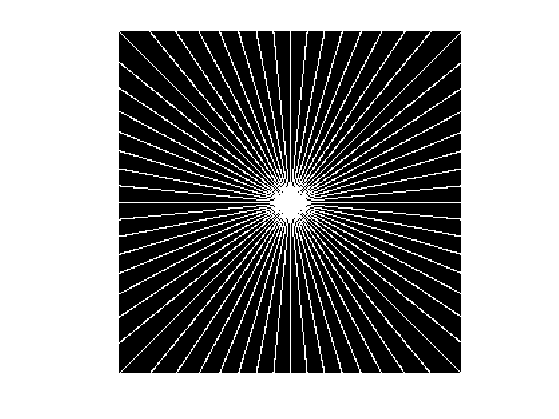Sampling the Fourier transform for points is equivalent to masking the Fourier transform $$\hat f$$ of $$f$$. We thus define our Fourier tomography measurements operator as $\Phi f(\omega) = \hat f(\omega) \xi(\omega)$ where $$\hat f$$ is the 2-D discrete Fourier transform of $$f$$.

Define a shortcut for the operator. Note the normalization of the FFT by $$1/n$$ to make it orthonormal.

Phi  = @(f)fft2(f).*xi / n;


Load the clean high resolution image.

name = 'mri';
f0 = rescale(f0);


We consider here noisy tomographic measurements. $y = \Phi f_0 + w$ where $$w$$ is a Gaussian white noise of variance $$\sigma^2$$.

Variance of the noise.

sigma = .2;


Measurements.

y = Phi(f0) + sigma*randn(n,n);


The pseudo inverse $$\Phi^+$$ is equal to the transposed operator $$\Phi^*$$. It corresponds to inverting the Fourier transform after multiplication by the mask. $\Phi^* y = \Phi^+ y = \mathcal{F}^{-1}( y \xi ),$ where $$\mathcal{F}^{-1}(\hat f) = f$$ is the inverse Fourier transform.

Define a shortcut for the transpose operator.

PhiS = @(y)real(ifft2(y.*xi))*n;


Exercice 1: (check the solution) Compute and display the pseudo inverse reconstruction $$\Phi^+ y$$. What do you observe ?

exo1;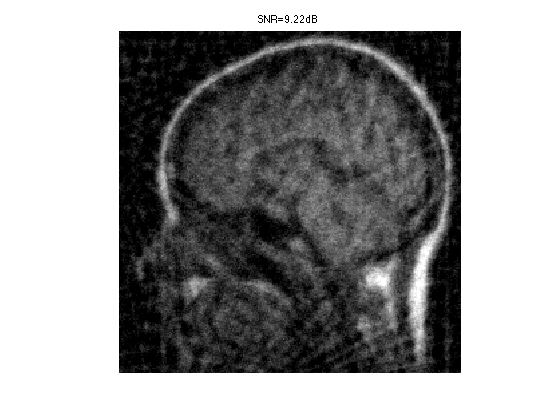## Sobolev Regularization

To remove some noise while inverting the operator, we can penalize high frequencies using Sobolev regularization.

The Sobolev prior reads: $J(f) = \frac{1}{2} \sum_x \|\nabla f(x)\|^2 = \frac{1}{2}\sum_{\omega} S(\omega) \|\hat f(\omega)\|^2$ where $$S(\omega)=\|\omega\|^2$$.

Compute the Sobolev prior penalty $$S$$ (rescale to $$[0,1]$$).

x = [0:n/2-1, -n/2:-1];
[Y,X] = meshgrid(x,x);
S = (X.^2 + Y.^2)*(2/n)^2;


We need to compute the solution of: $f^\star \in \text{argmin}_f \frac{1}{2}\|y-\Phi f\|^2 + \lambda J(f)$

Regularization parameter $$\lambda$$:

lambda = 2;


Since both the prior $$J$$ and the operator $$\Phi$$ can be written over the Fourier domain, one can compute the solution to the inversion with Sobolev prior simply with the Fourier coefficients: $\hat f^\star(\omega) = \frac{\hat y(\omega) \xi(\omega)}{ \xi(\omega) + \lambda S(\omega) }$

Perform the inversion.

fSob = real( ifft2( y .* xi ./ ( xi + lambda*S) ) )*n;


Display the result.

clf;
imageplot(clamp(fSob), ['Sobolev inversion, SNR=' num2str(snr(f0,fSob),3) 'dB'] );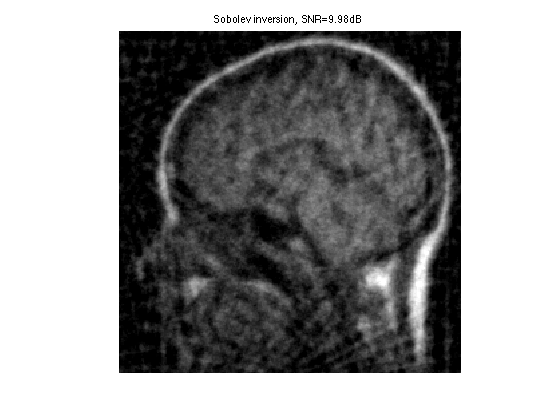Exercice 2: (check the solution) Find the optimal solution fSob by testing several value of $$\lambda$$.

exo2;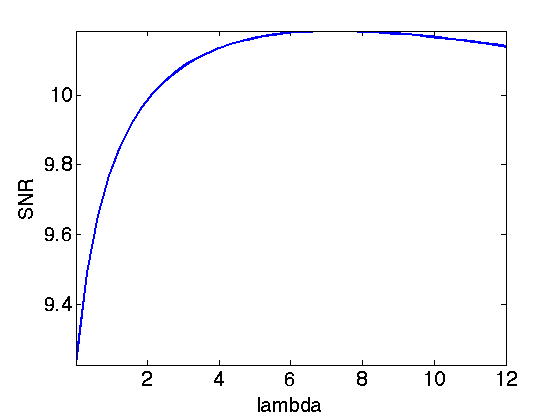Display optimal result.

clf;
imageplot(clamp(fSob), ['Sobolev inversion, SNR=' num2str(snr(f0,fSob),3) 'dB']);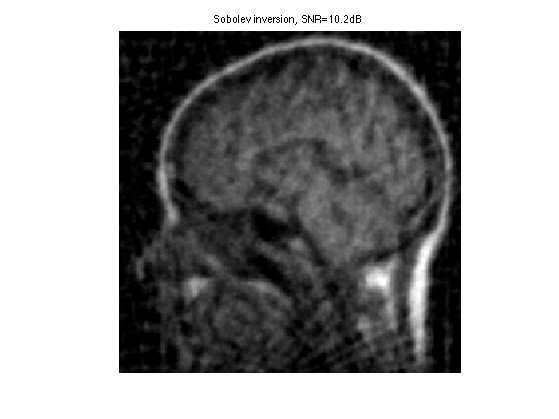## Soft Thresholding in a Basis

The soft thresholding operator is at the heart of $$\ell^1$$ minimization schemes. It can be applied to coefficients $$a$$, or to an image $$f$$ in an ortho-basis.

The soft thresholding is a 1-D functional that shrinks the value of coefficients. $s_T(u)=\max(0,1-T/|u|)u$

Define a shortcut for this soft thresholding 1-D functional.

SoftThresh = @(x,T)x.*max( 0, 1-T./max(abs(x),1e-10) );


Display a curve of the 1D soft thresholding.

clf;
T = linspace(-1,1,1000);
plot( T, SoftThresh(T,.5) );
axis('equal');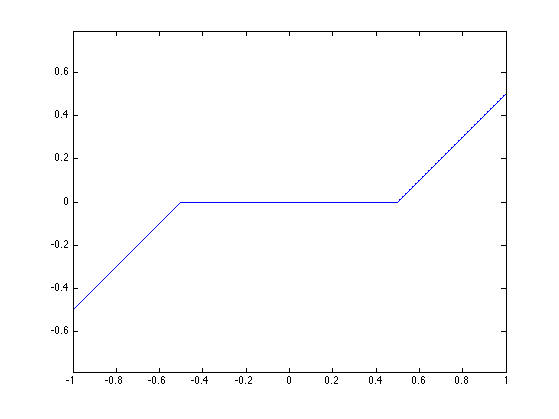Note that the function SoftThresh can also be applied to vector (because of Matlab/Scilab vectorialized computation), which defines an operator on coefficients: $S_T(a) = ( s_T(a_m) )_m.$

In the next section, we use an orthogonal wavelet basis $$\Psi$$.

We set the parameters of the wavelet transform.

Jmax = log2(n)-1;
Jmin = Jmax-3;


Shortcut for $$\Psi$$ and $$\Psi^*$$ in the orthogonal case.

options.ti = 0; % use orthogonality.
Psi = @(a)perform_wavelet_transf(a, Jmin, -1,options);
PsiS = @(f)perform_wavelet_transf(f, Jmin, +1,options);


The soft thresholding opterator in the basis $$\Psi$$ is defined as $S_T^\Psi(f) = \sum_m s_T( \langle f,\psi_m \rangle ) \psi_m$

It thus corresponds to applying the transform $$\Psi^*$$, thresholding the coefficients using $$S_T$$ and then undoing the transform using $$\Psi$$. $S_T^\Psi(f) = \Psi \circ S_T \circ \Psi^*$

SoftThreshPsi = @(f,T)Psi(SoftThresh(PsiS(f),T));


This soft thresholding corresponds to a denoising operator.

clf;
imageplot( clamp(SoftThreshPsi(f0,.1)) );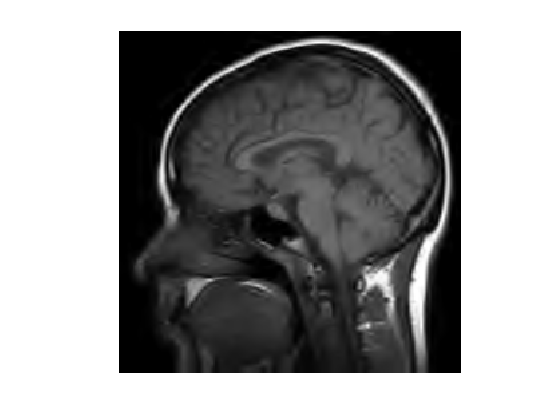## Sparse Regularization

To better reconstruct edges, we consider a sparsity regularization in an orthogonal basis $$\{ \psi_m \}_m$$: $J(f)=\sum_m \vert \langle f,\psi_m \rangle\vert = \| \Psi^* f \|_1.$

The inverse problem resolution thus require to solve: $f^{\star} \in \text{argmin}_f \: E(f) = \frac{1}{2}\|y-\Phi f\|^2 + \lambda \sum_m \vert \langle f,\psi_m \rangle\vert.$

Set up the value of the regularization parameter $$\lambda$$.

lambda = 0.1;


To solve this non-smooth optimization problem, one can use forward-backward splitting, also known as iterative soft thresholding.

It computes a series of images $$f^{(\ell)}$$ defined as $f^{(\ell+1)} = S_{\tau\lambda}^{\Psi}( f^{(\ell)} - \tau \Phi^{*} (\Phi f^{(\ell)} - y) )$

For $$f^{(\ell)}$$ to converge to a solution of the problem, the gradient step size should be chosen as $\tau < \frac{2}{\|\Phi^* \Phi\|}$

Since $$\Phi$$ is an operator of norm 1, this must be smaller than 2.

tau = 1.5;


Initialize the solution.

fSpars = PhiS(y);


First step: perform one step of gradient descent of the energy $$\|y-\Phi f\|^2$$.

fSpars = fSpars + tau * PhiS( y-Phi(fSpars) );


Second step: denoise the solution by thresholding.

fSpars = SoftThreshPsi( fSpars, lambda*tau );


Exercice 3: (check the solution) Perform the iterative soft thresholding. Monitor the decay of the energy $$E$$ you are minimizing.

exo3;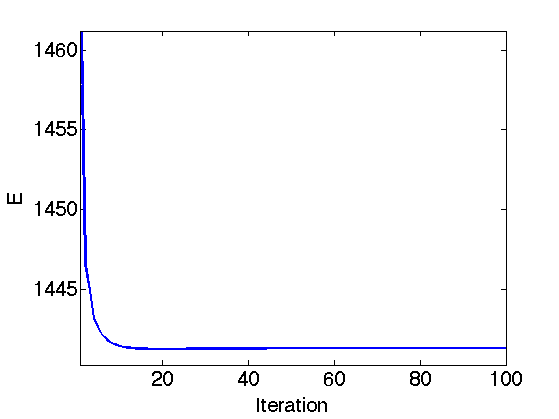Display the result.

clf;
imageplot(clamp(fSpars), ['Sparsity inversion, SNR=' num2str(snr(f0,fSpars),3) 'dB']);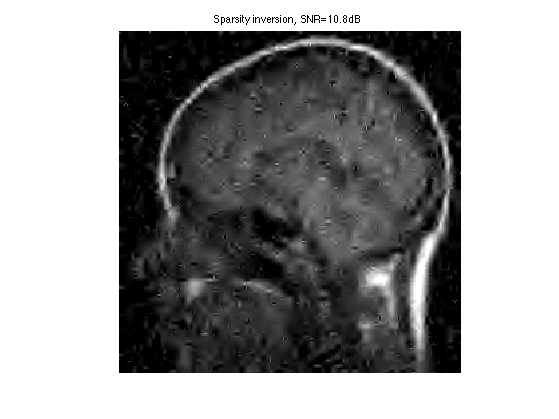Exercice 4: (check the solution) Try to find the best threshold $$\lambda$$. To this end, perform a lot of iterations, and progressively decay the threshold $$\lambda$$ during the iterations. Record the best result in fBestOrtho.

exo4;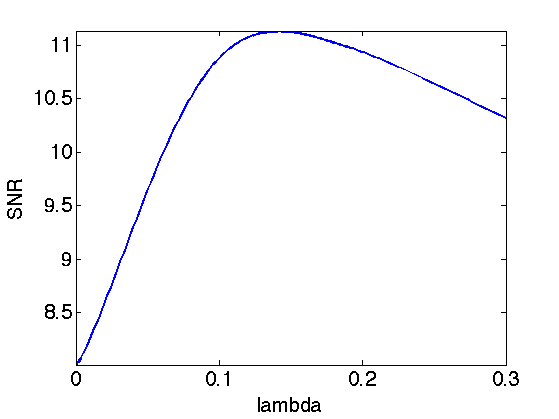Display the result.

clf;
imageplot(clamp(fBestOrtho), ['Sparsity in Orthogonal Wavelets, SNR=' num2str(snr(f0,fBestOrtho),3) 'dB']);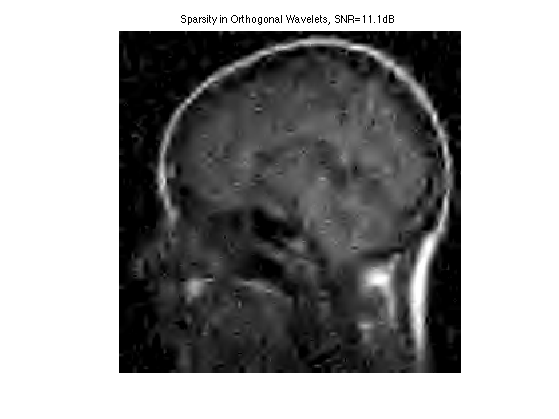## Translation Invariant Regularization

Denoising artefact are reduced by using a translation invariant wavelet frame.

Define new shortcut for the translation invariant wavelet transforms.

options.ti = 1;
Psi = @(a)perform_wavelet_transf(a, Jmin, -1,options);
PsiS = @(f)perform_wavelet_transf(f, Jmin, +1,options);


Re-define the thresholding.

SoftThreshPsi = @(f,T)Psi(SoftThresh(PsiS(f),T));


Exercice 5: (check the solution) Use the iterative thresholding but this time with the translation invariant wavelet transform. Find the best value of $$\lambda$$ and record the best result in fBestTI.

exo5;Display the result.

clf;
imageplot(clamp(fBestTI), ['Sparsity in TI Wavelets, SNR=' num2str(snr(f0,fBestTI),3) 'dB']);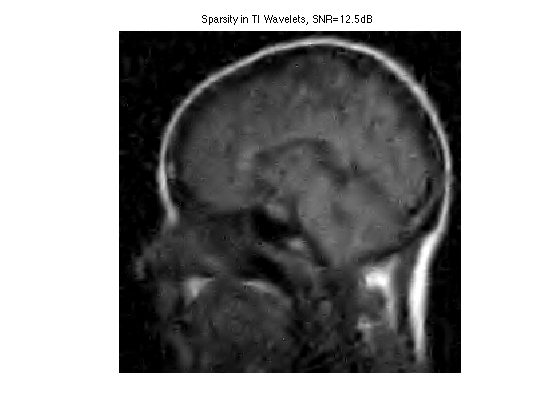## Medical Resonnance Imaging

MRI acquisition directly gather Fourier measurement along a curve in the Fourier plane.

Create a spiral for the sampling. The parameter $$\alpha$$ controls the density near the origin.

alpha = 3;
q = 30;
t = linspace(0,1.5,n*q*10);
x = round(.5*n*t.^alpha.*cos(2*pi*q*t)) + n/2+1;
y = round(.5*n*t.^alpha.*sin(2*pi*q*t)) + n/2+1;
I = find(x>0 & x<=n & y>0 & y<=n); x = x(I); y = y(I);
xi = zeros(n,n); xi(x+(y-1)*n) = 1;


Display the sampling pattern.

clf;
imageplot(xi);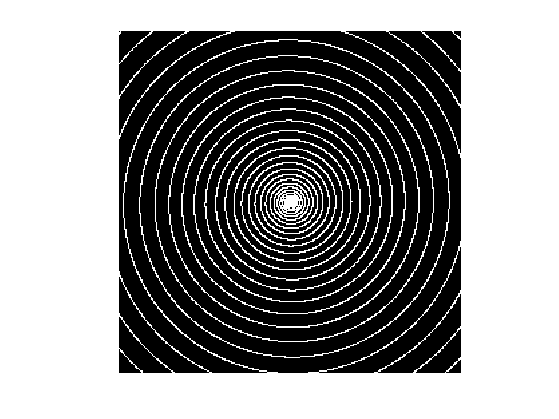Exercice 6: (check the solution) Compare Sobolev and Sparse reconstruction for MRI imaging. For a given number of Fourier sample, compare the quality of the reconstruction for different $$\alpha$$.

exo6;